# Newcastle MathsJam December 2011 Recap

Amazingly, December’s MathsJam had a non-trivial attendance of six whole people. And not just any people! Puzzling heavyweight David Cushing had yet more Renaissance-era riddles to test us all, and the other regulars were in similarly bamboozling form.

I balanced things out by failing to prepare anything or bringing anything to take notes on and subsequently forgetting most of what the others talked about. So this isn’t going to be a very accurate record of what happened, unless I get some reminders in the comments.

I’m going to start with a rather lengthy deconstruction of a puzzle Matthew Taylor posed:

## A “lights out” puzzle

Matthew posted this puzzle on twitter a couple of days before the MathsJam night. That could be considered either a tactical error or a helpful advance-warning because by the time we were all gathered together I had a rather thorough solution and explanation.

The set-up is that we have a $$3 \times 3$$ board, with cells that can be coloured either black or white. We can perform the following three types of move on the board:

1. Change the colour of each cell in one of the rows.
2. Change the colour of each cell in one of the columns.
3. Change the colour of each cell in one of the two diagonals.

Question 1: For any arrangement of the cells, is there a sequence of moves which will leave all the cells the same colour? If not, why not?

First of all, note that if the board is all white, then flipping all three rows will turn the board all black, and vice versa. Hence, an arrangement can be made all black if and only if it can be made all white, so it’s sufficient to show that each arrangement has a sequence of moves which result in the board being all-white.

Now give names to the moves: $$R_{1}$$,$$R_{2}$$,$$R_{3}$$ for the row moves, $$C_{1}$$,$$C_{2}$$,$$C_{3}$$ for the column moves and $$D_{1}$$ and $$D_{2}$$ for the diagonals.

Any move, applied twice in a row, leaves the board unchanged. Also, the moves all commute – it doesn’t matter what order you do them in.

So each move is either used once or not at all, and the order doesn’t matter.

There are $$8$$ moves, so there are at most $$2^{ 8 }$$ sequences producing different outcomes.

But there are $$9$$ cells on the board, so $$2^{ 9 }$$ possible arrangements, and every arrangement has a different solution sequence.

So straight away, the answer to the question is no: there are some arrangements which can’t be changed to be all the same colour using the moves given.

Question 2: Extend to $$N \times N$$ grids, with the logical extension of the rules.

An $$N \times N$$ grid has $$N^{ 2 }$$ cells but $$2 N + 2$$ moves. By the argument from Question $$1$$, a grid definitely has unsolvable arrangements when there are more cells than moves, i.e. $$N^2 \gt 2N+2$$.

$$N^{ 2 } = 2 N + 2$$ when $$N = 1 + \sqrt3 \approx 2.7$$.

So grids bigger than $$3 \times 3$$ are definitely unsolvable. A $$1 \times 1$$ grid is, of course, already solved by definition.

But what about $$2 \times 2$$?

Question 2(a): How many arrangements really can be “solved” with the given moves?

The answer to this one isn’t necessarily $$2^{2N+2}$$: if one of the moves can be reproduced using some combination of the other moves, then we don’t have as many degrees of freedom as we thought.

We can immediately get rid of one of the column moves: flip all the rows, so every cell has been changed. Now flip all the columns except one. The flipped columns are back to how they started, and all the cells in the remaining column have changed.

What I’m really trying to do in this question is to find an independent basis (or generating) set for the given moves. You can think about the set of arrangements of a board as a group — as well as representing the colouring of the board, an arrangement can also represent a set of cells to flip. I like this idea because I’m a group theorist.

So when you apply one arrangement to another, each cell in the resulting arrangement is black if and only if it was black in exactly one of the original arrangements. This is functionally the same as the $$\operatorname{XOR}$$ operation, or addition modulo $$2$$.

Hence, the set of arrangements that can be solved is the same as the set of arrangements that can be produced using the moves we’re given.

At this point I broke out Python and did a bit of programming. This code is the result. It asks for the size of the board, then it works out how many arrangements can be produced by the given moves and hence how many independent moves there are.

For the $$3 \times 3$$ grid, there are $$7$$ independent generators, producing $$128$$ different arrangements, and for the $$2 \times 2$$ grid, there are 3 generators producing $$8$$ different arrangements. So the $$2 \times 2$$ grid isn’t solvable with the given moves, either!

Question 3: If there are unsolvable arrangements – given any two, can we connect them by a series of moves?

For $$3 \times 3$$ grids, the answer is no, because we’re missing $$2$$ independent generators. For the $$2 \times 2$$ grid, the answer is yes. Because I’ve spent all afternoon writing up just this puzzle, I’ll leave it up to you to see why and how.

OK, that went on a bit longer than I intended. It’s a good puzzle though, and I used it as an example of applied group theory for a friend doing a third-year algebraic structures course whose lecturer hadn’t seen fit to show him any groups that weren’t $$\mathbb{Z}^n$$ or $$\mathbb{Z}_n$$, or even any applications of those to real problems! He was having trouble understanding what quotient groups are all about so, since we’ve just generated a nice normal subgroup of the group of arrangements of the board, I showed him what you get when you quotient it out: fun times!

On the MathsJam night, I guided the others to this solution with a few hints and nudges, and a grid of pennies representing the board.

Matthew asked where would be a good place to do a PhD in maths, preferably a project on something to with braid groups. It was agreed that funded PhDs are thin on the ground these days, and David (part-time funded) and I (self-funded) weren’t optimistic about the chances of Matthew getting funding at Newcastle, much as we’d like to have him around.

I’m not completely sure who gave this next puzzle, but I think it might have been Matthew again:

## A picky-interval game

$$A$$ and $$B$$ play a game. They draw out the interval $$[0,1]$$ and pick a point $$x$$ inside it. They take turns choosing sub-intervals as follows:

1. each player’s interval has to be contained entirely in the previous player’s interval
2. $$A$$‘s interval must be exactly $$\alpha \in [0,1]$$ as big as $$B$$‘s previous one. Similarly, $$B$$‘s interval has to be exactly $$\beta$$ times as big as $$A$$‘s previous one.

If $$B$$ picks an interval not containing the point $$x$$, then $$B$$ wins. Otherwise, if after infinitely many goes $$B$$ hasn’t won, then $$A$$ wins. Player $$A$$ must have patience in abundance for this game.

I won’t write out a solution because frankly I’m brain-drained from writing up the lights-out puzzle. Anyway, it was rather satisfying to see how the game worked, and David and I did a lot of scribbling: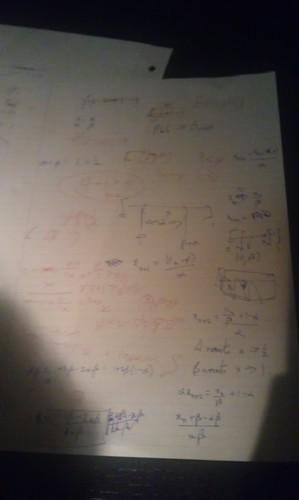Talking of David, he followed up his excellent princess-on-a-graph puzzle with one about a knight and a dragon:

## A knight, a dragon and six poisoned wells.

A knight and a dragon have a fight which ends in a draw. They agree to settle the matter with a battle of wits. They go to a place with a freshwater pond and six poisoned wells. The first five wells are accessible to both the knight and the dragon, but the sixth well is on a high promontory that only the dragon can reach.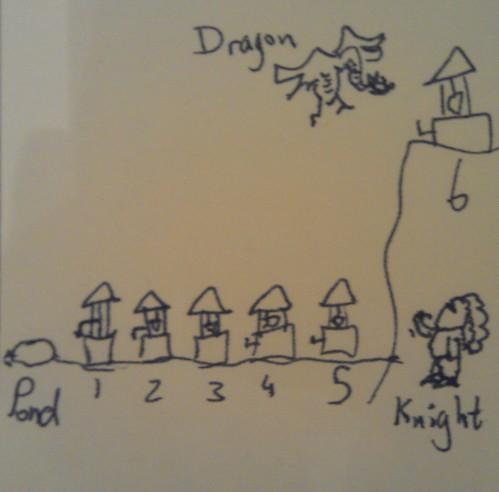Each well is poisoned but the poison can be cancelled out by drinking water from a well with a bigger number. So if somebody drinks from well $$1$$ and then from well $$2$$ they will survive, but if they drink from well $$2$$ and then well $$1$$, they will still be poisoned and will die the next day.

The dragon and the knight agree to spend the night concocting their strategies, and in the morning they will each go in secret to fetch some water to give to each other.

What happens?

Your ideas, please! I didn’t get the solution without a lot of hints from David.

I also have written down something about a “train puzzle”. I can’t remember what it was. If it was David’s train puzzle, maybe he can talk about it in the comments.

David brought his collection of Rubik’s cube-ish toys: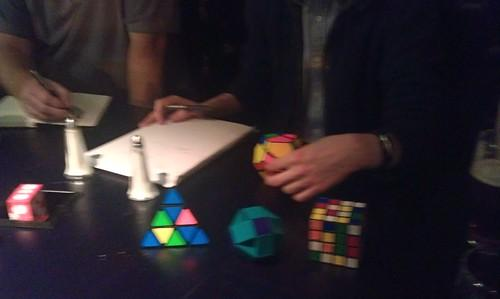The pyraminx (the pyramid-shaped one) was the only one I could get my head round. The dodecahedron one was far too hard.

Eamonn brought some puzzling blocks which we were supposed to fit together.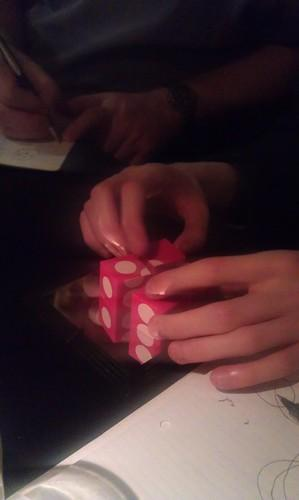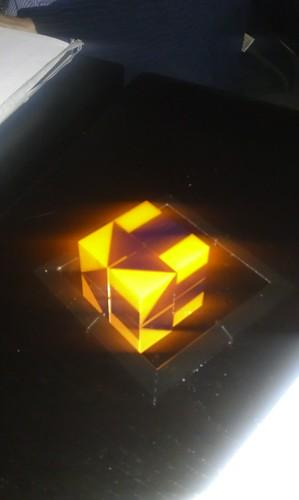Andrew Lobb pointed out on twitter later on that the one with numbers on isn’t a real die because $$4$$ and $$3$$ should be opposite each other.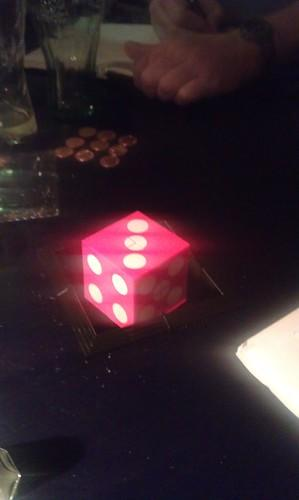Dan Woodhouse asked: Assign numbers $$1 \dots N$$ to the vertices of a tree of $$N$$ vertices. Label the edges with the difference between the numbers at their ends. Can all edges have unique labels?

I had a good sporting go at it until he told me the question is famously open. Oh well.

A rectangle is divided up into several smaller rectangles. For each smaller rectangle, either the horizontal or vertical sides have integer length. Is it true that the large rectangle must have sides of integer length?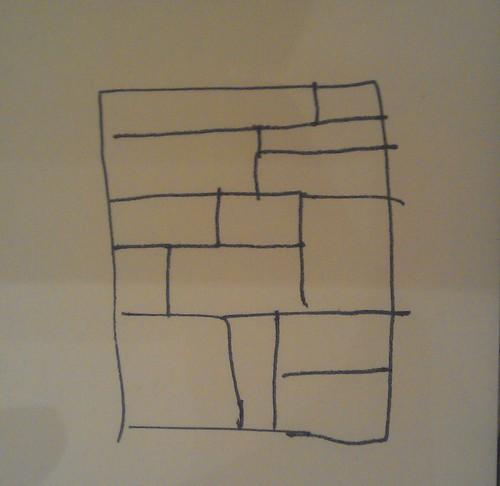The answer is yes and John gave three different proofs, all of which I failed to write down. One of them involved integrating $$e^{2\pi(x+y)}$$ across the whole rectangle.

I have a few other things written down that I can’t fully remember:

• Something about the random number generator in Tetris
• What’s the point in maths?
• Maths is art — I can’t remember if the contention was that maths should be considered alongside the arts subjects instead of the sciences, or whether it was something to do with how elegance or “beauty” are desirable goals in mathematics. Anyway, I definitely remember taking the opportunity to plug my arty maths blog.
• Is there a 3d version of buffon’s needle?
• What fraction of all triangles are acute? This question was discussed by Richard Guy in an essay entitled, “There are three times as many obtuse-angled triangles as acute-angled ones.” No prizes for guessing the answer, but the essay gives five different proofs of the headline value and a few dissenting answers. It’s part of the excellent anthology “Is Mathematics Inevitable?” which I must remember to bring along to MathsJam at some point.

So that was December’s MathsJam. Maybe in January I’ll be a bit better-prepared and take better notes.

By the way, I wrote up our solution to the princess-on-a-graph puzzle last month in a post that has been woefully under-appreciated, judging by the visitor stats. Rectify that, if you would be so kind.

The train problem is as follows. There is an infinite train track with a train travelling somewhere along the track at an unknown speed with an unknown location. There are sensor posts right along the track placed 1m apart from each other. When a post is activated it will detect if the train passes through it within the next second.

Does there exist a strategy to catch the train?

I forgot to mention that you can activate 1 post every second and you do not know what direction the train is travelling in.

Nice writeup! A few things to help out;

— My friend from Durham was called Dan Woodhouse if you feel like changing that (but I’m sure he won’t mind).

— It may be worth writing up a little about that train problem… the solution is so mindblowing I’m sure it’s probably illegal in several countries. David had trouble convincing me it worked. 🙂

— We also discussed the Melbourne Puzzle Hunt; Christian suggested getting a team together for that. There was mixed reaction given the difficulty of the puzzles from the last one!

— The interval question is a one-dimensional version of something called Schmidt’s Game. It sadly doesn’t have a Wikipedia page (Schmidt himself only gets 5 lines) but it was something I found during my research into Diophantine approximation for a PhD application.

Apparently Schmidt used it to prove that the set of badly approximable vectors in N-dimensional Euclidean space has full Hausdorff dimension. Nope, me neither. But the game itself is nicely understandable. The impression I get from the papers is that Schmidt generalised it to an N-dimensional ball (which I don’t think would take much more work – see below).

Anyway, David and Christian came up with a pretty cool way of thinking about it not mentioned here, which is like knocking a penny backwards and forwards along the width of a table; the ratios correspond, in a manner of speaking, to how far each player is allowed to push the penny in this analogy and in my opinion it’s a much easier way of understanding why a solution works. This also presumably allows you to generalise to N dimensions easily because if the penny falls off any of the N tables then B wins? I think. That’s just a gut feeling, I’m not sure if I’m right.

— John’s integral proof of that rectangle thing was fantastic. Integrating $e^{2 \pi i}(x + y)$ over any rectangle gives zero if and only if at least one side has integer length. Sum the integrals over each small rectangle and you’re done.

That’s all I can think of for now. 🙂

Thanks for those reminders Matthew. I’ve given Dan due credit in the post.

One thing though: I certainly did not suggest getting a team together for the Melbourne Puzzle Hunt! David is keen but I am definitely not.

Ah, of course! I remember now. That would explain why you didn’t write it up. 🙂

And a simple error in my comment; Dan is from Warwick, not Durham.

The rectangles problem and its solutions came from Proofs From THE BOOK.

Proof 1: integrate $e^{2 \pi i(x+y)}$ over an axis-aligned rectangle. The result is zero if and only if the rectangle has an integer edge (you can prove that yourselves. One direction is trivial, the other merely easy). The integral over the large rectangle is the sum of the integrals over the small ones, which is zero.

Proof 2: is related. Overlay a checkerboard pattern with $\frac{1}{2} \times \frac{1}{2}$ squares. A rectangle with an integer side has equal areas of black and white, so the large one does too. If the large one is $x$ by $y$, slice off everything to the left of $\lfloor x \rfloor$ and below $\lfloor y \rfloor$. The remainder (less than a $1 \times 1$ square) will have more of one colour unless at least one edge is zero length.

Proof 3: Put a black vertex in the centre of each small rectangle and a white vertex on each corner that has both coordinates integer. Join each black vertex to any white vertices on the corners of its rectangle (if any). Then each black vertex has an even number of edges, so there is an even number of edges in total. White vertices that aren’t on the corners of the large rectangle also have an even number of edges. There is a white vertex at $(0,0)$ with only one edge, so there must be at least one more white vertex on a corner of the large rectangle.

Ah, I have that book! Shows how much attention I paid when reading it. I’ve TeXed your comment to make the maths look nice, hope you don’t mind. Thanks for writing out the proofs.

I also had a look at the tree-labelling problem, and not surprisingly got almost nowhere.

Trivial observations: given one tree, you can make a larger one by adding a new vertex adjecent to 0. You can also negate all the vertices mod N without changing the edge labels.

I was hoping that there would be a way of renumbering a tree to move the 0 to any position, which would make it easy. But no luck: there are trees which contain vertices that can never be 0.

Curiously, if you add a new vertex adjacent to one that can’t be 0, it changes status – now it can be 0 (the one you added can’t). At least, it does in the trees I’ve looked at.

I have a complete set of solutions for trees up to 8 vertices, and possible locations of 0 for trees up to 10. I’ll bring it along next time if anyone is interested.

Have you now decided not to follow through with your dastardly scheme to entrap a princess on the train?

I never followed through about worries whether the train had an integer speed or not, and whether that made a difference to the strategy.

If you assume integer speed you can generalise the solution to any speed. There are two easier versions of the puzzle
(i) You know that the train is travelling at an unkown integer speed and where it was a second ago.
(ii) you know the trains integer speed and direction it is travelling. You also know a point at which the train has passed.
Problem two is like princess in a castle but distracted me from how to solve the full problem. The first one can be solved in a way that generalises to a full solution quite easily. I didn’t know this for months

I’ve only just read that it was you who posted that Alastair so you know the solution. Michael white said since the rationals are dense in the reals we can take any speed. We are dealing with a knight catching a dragon now instead of princesses. There is also a new problem.
There is a circular area with a dragon in it. We fire a catapult of fire T the dragon which is a circle of radius c. If the fire hits the dragon we win. Otherwise the fire is put out and the dragon moves d inside the circle. Under what c,d can we catch the dragon?

I’m not convinced that the train can have any (real) speed. The solution I have in mind works for rational speed, but the extension to reals is not obvious. Because the rationals are countable, we can iterate over pairs of rationals. Each pair gives a starting position and speed to test, and then we just have to look at the section of track that the train would occupy at this time.
You could say that there are rationals as close to any given real as you like, but that’s not enough. The difference between the two speeds gets multiplied by time, so you need to visit that pair before the difference becomes greater than 1.
In the simplest version of my solution, you can’t visit a speed with denominator q before time $4q^4$. That can be improved, but it will always grow faster than $q^2$.
The best rational approximations to a real are the convergents of the continued fraction, and the error is proportional to $1/q^2$. We need to visit this rational before $t/Cq^2 > 1$, which means $t < Cq^2$. But that can't happen.
Have I got the wrong solution? Or is there a subtlety that I'm missing?